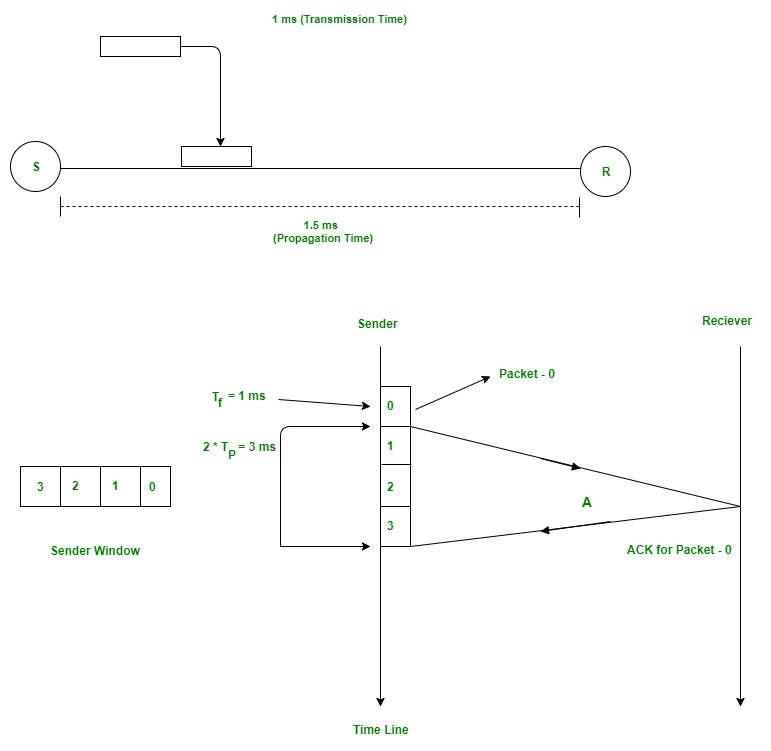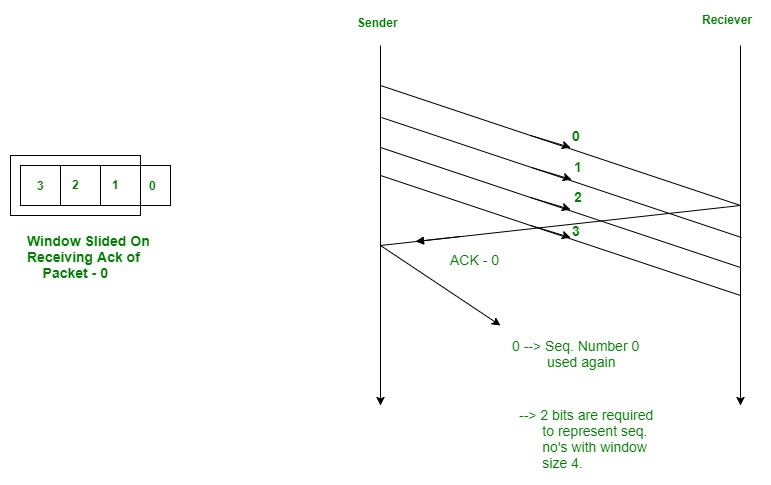# Sliding Window Protocol | Set 1 (Sender Side)

Prerequisite : Stop and Wait ARQ

The Stop and Wait ARQ offers error and flow control, but may cause big performance issues as sender always waits for acknowledgement even if it has next packet ready to send. Consider a situation where you have a high bandwidth connection and propagation delay is also high (you are connected to some server in some other country though a high speed connection), you can’t use this full speed due to limitations of stop and wait.

Sliding Window protocol handles this efficiency issue by sending more than one packet at a time with a larger sequence numbers. The idea is same as pipelining in architectures.

## Few Terminologies :

Transmission Delay (Tt) – Time to transmit the packet from host to the outgoing link. If B is the Bandwidth of the link and D is the Data Size to transmit

`    Tt = D/B  `

Propagation Delay (Tp) – It is the time taken by the first bit transferred by the host onto the outgoing link to reach the destination. It depends on the distance d and the wave propagation speed s (depends on the characteristics of the medium).

`   Tp = d/s  `

Efficiency – It is defined as the ratio of total useful time to the total cycle time of a packet. For stop and wait protocol,

```Total cycle time = Tt(data) + Tp(data) +
Tt(acknowledgement) + Tp(acknowledgement)
=  Tt(data) + Tp(data) + Tp(acknowledgement)
=   Tt + 2*Tp
```

Since acknowledgements are very less in size, their transmission delay can be neglected.

```Efficiency = Useful Time / Total Cycle Time
= Tt/(Tt + 2*Tp) (For Stop and Wait)
= 1/(1+2a)  [ Using a = Tp/Tt ]
```

Effective Bandwidth(EB) or Throughput – Number of bits sent per second.

```EB = Data Size(L) / Total Cycle time(Tt + 2*Tp)
Multiplying and dividing by Bandwidth (B),
=  (1/(1+2a)) * B   [ Using a = Tp/Tt ]
=  Efficiency * Bandwidth
```

Capacity of link – If a channel is Full Duplex, then bits can be transferred in both the directions and without any collisions. Number of bits a channel/Link can hold at maximum is its capacity.

``` Capacity = Bandwidth(B) * Propagation(Tp)

For Full Duplex channels,
Capacity = 2*Bandwidth(B) * Propagation(Tp)
```

## Concept Of Pipelining

In Stop and Wait protocol, only 1 packet is transmitted onto the link and then sender waits for acknowledgement from the receiver. The problem in this setup is that efficiency is very less as we are not filling the channel with more packets after 1st packet has been put onto the link. Within the total cycle time of Tt + 2*Tp units, we will now calculate the maximum number of packets that sender can transmit on the link before getting an acknowledgement.

``` In Tt units ----> 1 packet is Transmitted.
In 1 units  ----> 1/Tt packet can be Transmitted.
In  Tt + 2*Tp units ----->  (Tt + 2*Tp)/Tt
packets can be Transmitted
------>  1 + 2a  [Using a = Tp/Tt]
```

Maximum packets That can be Transmitted in total cycle time = 1+2*a

Let me explain now with the help of an example.

Consider Tt = 1ms, Tp = 1.5ms.

In the picture given below, after sender has transmitted packet 0, it will immediately transmit packets 1, 2, 3. Acknowledgement for 0 will arrive after 2*1.5 = 3ms. In Stop and Wait, in time 1 + 2*1.5 = 4ms, we were transferring one packet only. Here we keep a window of packets which we have transmitted but not yet acknowledged.After we have received the Ack for packet 0, window slides and the next packet can be assigned sequence number 0. We reuse the sequence numbers which we have acknowledged so that header size can be kept minimum as shown in the diagram given below.## Minimum Number Of Bits For Sender window (Very Important For GATE)

As we have seen above,

``` Maximum window size = 1 + 2*a    where a = Tp/Tt

Minimum sequence numbers required = 1 + 2*a. ```

All the packets in the current window will be given a sequence number. Number of bits required to represent the sender window = ceil(log2(1+2*a)).

But sometimes number of bits in the protocol headers is pre-defined. Size of sequence number field in header will also determine the maximum number of packets that we can send in total cycle time. If N is the size of sequence number field in the header in bits, then we can have 2N sequence numbers.

Window Size ws = min(1+2*a, 2N)

If you want to calculate minimum bits required to represent sequence numbers/sender window, it will be ceil(log2(ws)).

In this article, we have discussed sending window only. For receiving window, there are 2 protocols namely Go Back N and Selective Repeat which are used to implement pipelining practically. We will be discussing receiving window in set 2.